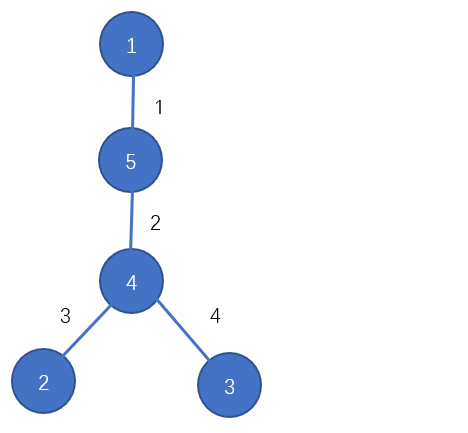# 2023 年上海市大学生程序设计竞赛 - 一月赛

F. Hide and seek

lbromine,KomorebiandAmuzilike to play hide-and-seek in the teaching building. The teaching building is very large and its structure is very strange. It is composed of $n$ classrooms numbered from $1$ to $n$ and $n-1$ two-way passages, and any two classrooms can reach each other.

lbromineis responsible for finding and KomorebiandAmuziare responsible for hiding.But the teaching building is too big, solbromine makes a rule that the KomorebiandAmuzi can only hide in the classroom with the classroom number in the range of $[l, r]$.

At the beginning,lbrominecan use his transmission device to transmit to any classroom in the teaching building with $0$ seconds, and then search for Komorebiand Amuzialong the passages of the classrooms. In order to evaluate whether the selected interval $[l, r]$is reasonable, lbromine wants to know the shortest possible time he needs to find Komorebi and Amuzifor some intervals.

### 输入格式

The first line of input contains a positive integer $n$ ($1 \leq n\leq1 \times10 ^ 5$) , indicating the number of classrooms in the teaching building.

The next $n-1$ line has three integers $u, v, w$ in each line, indicating that there is a passage between classroom $u$ and classroom $v$ , and lbromine needs $w$ seconds to pass through the passage $(1 \leq u, v \leq n, 1 \leq w \leq 10 ^ 9)$

An integer $q$ in line $n+1$ represents the number of queries $(1 \leq q \leq1 \times 10 ^ 4)$

The next $q$ line has two integers $l,r$ per line,indicating the query interval $(1 \leq l<r \leq n)$

### 输出格式

For each query output one line contains one integer indicating the shortest possible time for him to find Komorebi and Amuzi.

### 样例

Input
5
1 5 1
5 4 2
2 4 3
3 4 4
3
1 3
3 5
2 3

Output
6
2
7


### 提示

The structure of the teaching building in the sample is as followsSo for the interval $[1,3]$ ,if Komorebi hides in classroom $1$ and Amuzi hides in classroom $2$,lbromine can transmit to classroom $1$ and go through classroom $5$ and $4$ to classroom $2$ using $6$ secends.It can be proved that this is the shortest possible time for lbromine to find Komorebi and Amuzi.

NaN